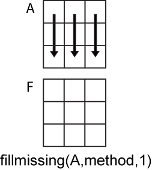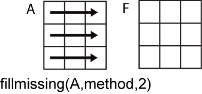# fillmissing

## 语法

``F = fillmissing(A,'constant',v)``
``F = fillmissing(A,method)``
``F = fillmissing(A,movmethod,window)``
``F = fillmissing(A,'knn')``
``F = fillmissing(A,'knn',k)``
``F = fillmissing(A,fillfun,gapwindow)``
``F = fillmissing(___,dim)``
``F = fillmissing(___,Name,Value)``
``[F,TF] = fillmissing(___)``

## 说明

````F = fillmissing(A,'constant',v)` 使用常量值 `v` 填充缺失的数组或表条目。如果 `A` 是矩阵或多维数组，则 `v` 可以是标量或向量。如果 `v` 是向量，则每个元素指定 `A` 的对应列中的填充值。如果 `A` 是表或时间表，则 `v` 也可以是元胞数组，其元素包含每个表变量的填充值。缺失值的定义取决于 `A` 的数据类型： `NaN` - `double`、`single`、`duration` 和 `calendarDuration``NaT` — `datetime``<missing>` — `string``<undefined>` — `categorical``{''}` - 字符向量的 `cell` 如果 `A` 是表，则每个变量的数据类型定义该变量的缺失值。```

``F = fillmissing(A,method)` 使用 `method` 指定的方法填充缺失的条目。例如，`fillmissing(A,'previous')` 对 `A` 中的缺失条目使用上一个非缺失条目进行填充。`

``F = fillmissing(A,movmethod,window)` 使用窗长度为 `window` 的移动窗均值或中位数填充缺失条目。例如，`fillmissing(A,'movmean',5)` 使用窗长度为 5 的移动均值填充缺失数据。`

``F = fillmissing(A,'knn')` 用最近邻行中的对应值填充缺失条目，这些值是根据行之间的成对欧几里德距离计算的。`
``F = fillmissing(A,'knn',k)` 用 `k` 最近邻行中对应值的均值填充缺失条目，该均值是根据行之间的成对欧几里德距离计算的。例如，`fillmissing(A,'knn',5)` 用五个最近邻行中对应值的均值填充 `A` 中的缺失条目。`

``F = fillmissing(A,fillfun,gapwindow)` 使用由函数句柄 `fillfun` 指定的自定义方法填充缺失条目的空缺，并在每个空缺周围设置固定窗，从该窗计算填充值。`fillfun` 必须具有输入参数 `xs`、`ts` 和 `tq`，它们是向量，分别包含长度为 `gapwindow` 的采样数据 `xs`、长度为 `gapwindow` 的采样数据位置 `ts` 和缺失数据位置 `tq`。`ts` 和 `tq` 中的位置是采样点向量的子集。`

``F = fillmissing(___,dim)` 支持上述语法中的任何输入参数组合，且可指定 `A` 中要进行运算的维度。默认情况下，`fillmissing` 沿其大小不为 1 的第一个维度进行运算。例如，如果 `A` 是矩阵，则 `fillmissing(A,2)` 跨 `A` 的各列进行运算，逐行填充缺失的数据。`

``F = fillmissing(___,Name,Value)` 使用一个或多个名称-值参数指定用于填充缺失值的其他参数。例如，如果 `t` 是时间值向量，则 `fillmissing(A,'linear','SamplePoints',t)` 会基于 `t` 中的时间值对 `A` 中的数据进行插值。`

``[F,TF] = fillmissing(___)` 还返回逻辑数组 `TF`，该数组指示 `F` 中先前缺失现已填充的条目的位置。`

## 示例

```A = [1 3 NaN 4 NaN NaN 5]; F = fillmissing(A,'previous')```
```F = 1×7 1 3 3 4 4 4 5 ```

`A = [1 NaN; NaN 2]`
```A = 2×2 1 NaN NaN 2 ```
`F = fillmissing(A,'constant',[100 1000])`
```F = 2×2 1 1000 100 2 ```

```x = [-4*pi:0.1:0, 0.1:0.2:4*pi]; A = sin(x);```

`NaN` 值插入 `A` 中。

`A(A < 0.75 & A > 0.5) = NaN;`

`[F,TF] = fillmissing(A,'linear','SamplePoints',x);`

```scatter(x,A) hold on scatter(x(TF),F(TF)) legend('Original Data','Filled Data')``````x = linspace(0,10,200); A = sin(x) + 0.5*(rand(size(x))-0.5); A([1:10 randi([1 length(x)],1,50)]) = NaN; ```

```F = fillmissing(A,'movmedian',10); plot(x,F,'.-') hold on plot(x,A,'.-') legend('Original Data','Filled Data')``````t = 10:10:100; A = [0.1 0.2 0.3 NaN NaN 0.6 0.7 NaN 0.9 1]; scatter(t,A)```• `xs` - 用于填充的数据值

• `ts` - 用于填充的值相对于采样点的位置

• `tq` - 缺失值相对于采样点的位置

• `n` - 要填充的空缺中的值的数目

```n = 2; gapwindow = [10 0]; [F,TF] = fillmissing(A,@(xs,ts,tq) forwardfill(xs,ts,tq,n),gapwindow,'SamplePoints',t);```

• `xs = 0.3`

• `ts = 30`

• `tq = [40 50]`

• `xs = 0.7`

• `ts = 70`

• `tq = 80`

```scatter(t,A) hold on scatter(t(TF),F(TF))``````function y = forwardfill(xs,ts,tq,n) % Fill n values in the missing gap using the previous nonmissing value y = NaN(1,numel(tq)); y(1:min(numel(tq),n)) = xs; end```

```A = [NaN NaN 5 3 NaN 5 7 NaN 9 NaN; 8 9 NaN 1 4 5 NaN 5 NaN 5; NaN 4 9 8 7 2 4 1 1 NaN]```
```A = 3×10 NaN NaN 5 3 NaN 5 7 NaN 9 NaN 8 9 NaN 1 4 5 NaN 5 NaN 5 NaN 4 9 8 7 2 4 1 1 NaN ```
`F = fillmissing(A,'linear',2,'EndValues','nearest')`
```F = 3×10 5 5 5 3 4 5 7 8 9 9 8 9 5 1 4 5 5 5 5 5 4 4 9 8 7 2 4 1 1 1 ```

```A = table(categorical({'Sunny'; 'Cloudy'; ''}),[66; NaN; 54],{''; 'N'; 'Y'},[37; 39; NaN],... 'VariableNames',{'Description' 'Temperature' 'Rain' 'Humidity'})```
```A=3×4 table Description Temperature Rain Humidity ___________ ___________ __________ ________ Sunny 66 {0x0 char} 37 Cloudy NaN {'N' } 39 <undefined> 54 {'Y' } NaN ```

`F = fillmissing(A,'previous')`
```F=3×4 table Description Temperature Rain Humidity ___________ ___________ __________ ________ Sunny 66 {0x0 char} 37 Cloudy 66 {'N' } 39 Cloudy 54 {'Y' } 39 ```

`A``Temperature``Humidity` 变量的 `NaN` 值替换为 0。

`F = fillmissing(A,'constant',0,'DataVariables',{'Temperature','Humidity'})`
```F=3×4 table Description Temperature Rain Humidity ___________ ___________ __________ ________ Sunny 66 {0x0 char} 37 Cloudy 0 {'N' } 39 <undefined> 54 {'Y' } 0 ```

`F = fillmissing(A,'constant',0,'DataVariables',@isnumeric)`
```F=3×4 table Description Temperature Rain Humidity ___________ ___________ __________ ________ Sunny 66 {0x0 char} 37 Cloudy 0 {'N' } 39 <undefined> 54 {'Y' } 0 ```

`F = fillmissing(A,'constant',{categorical({'None'}),1000,'Unknown',1000})`
```F=3×4 table Description Temperature Rain Humidity ___________ ___________ ___________ ________ Sunny 66 {'Unknown'} 37 Cloudy 1000 {'N' } 39 None 54 {'Y' } 1000 ```

```t = seconds([2 4 8 17 98 134 256 311 1001]); A = [1 3 23 NaN NaN NaN 100 NaN 233];```

`F = fillmissing(A,'linear','SamplePoints',t,'MaxGap',seconds(250))`
```F = 1×9 1.0000 3.0000 23.0000 25.7944 50.9435 62.1210 100.0000 NaN 233.0000 ```

```A = [1 3 9 3; -5 1 7 2; -1 1 7 NaN; 12 1 9 1]; m = isnan(A(3,:));```

```d1 = @(x,~) sum(abs(diff(x)),'omitnan'); dinf = @(x,isNaN) norm(diff(x(:,~isNaN(1,:))),'inf');```

`d1s = arrayfun(@(r) d1(A([r 3],:),m), setdiff(1:4,3))`
```d1s = 1×3 6 4 15 ```

`fillmissing` 函数将第三行中的 `NaN` 替换为第二行中对应的 `2`

`F1 = fillmissing(A,'knn','Distance',d1)`
```F1 = 4×4 1 3 9 3 -5 1 7 2 -1 1 7 2 12 1 9 1 ```

`dinfs = arrayfun(@(r) dinf(A([r 3],:),m), setdiff(1:4,3))`
```dinfs = 1×3 2 4 13 ```
`Finf = fillmissing(A,'knn','Distance',dinf)`
```Finf = 4×4 1 3 9 3 -5 1 7 2 -1 1 7 3 12 1 9 1 ```

## 输入参数

• 如果 `A` 为时间表，则仅填充表值。如果关联的行时间向量包含 `NaT``NaN` 值，则 `fillmissing` 会产生错误。行时间必须是唯一的并按升序列出。

• 如果 `A` 是元胞数组或包含元胞数组变量的表，则 `fillmissing` 仅在元胞数组包含字符向量时填充缺失元素。

• 如果 `A` 是矩阵或多维数组，则 `v` 可以是向量，表示每个运算维度的一个不同填充值。`v` 的长度必须与运算维度的长度相匹配。

• 如果 `A` 是表或时间表，则 `v` 可以是填充值的元胞数组，表示每个变量的一个不同填充值。元胞数组中的元素数必须与表中的变量数目相匹配。

`'previous'`上一个非缺失值
`'next'`下一个非缺失值
`'nearest'`距离最近的非缺失值
`'linear'`相邻非缺失值的线性插值（仅限数值、`duration``datetime` 数据类型）
`'spline'`分段三次样条插值（仅限数值、`duration``datetime` 数据类型）
`'pchip'`保形分段三次样条插值（仅限数值、`duration``datetime` 数据类型）
`'makima'`修正 Akima 三次 Hermite 插值（仅限数值、`duration``datetime` 数据类型）

`'movmean'`窗长度为 `window` 的移动均值（仅限数值数据类型）
`'movmedian'`窗长度为 `window` 的移动中位数（仅限数值数据类型）

`'knn'` 方法计算平均值的最近邻的数量，指定为正整数标量。

`xs`包含用于填充的数据值的向量。`xs` 的长度必须与指定窗的长度匹配。
`ts`包含用于填充的值的位置的向量。`ts` 的长度必须与指定窗的长度匹配。`ts` 是采样点向量的子集。
`tq`包含缺失值位置的向量。`tq` 是采样点向量的子集。

`A` 是时间表或 `SamplePoints` 指定为 `datetime``duration` 向量时，`gapwindow` 必须为 `duration` 类型。

• `fillmissing(A,method,1)` 根据 `A` 的每列中的数据填充缺失值，并返回一个 `m`×`n` 矩阵。• `fillmissing(A,method,2)` 根据 `A` 的每行中的数据填充缺失值，并返回一个 `m`×`n` 矩阵。### 名称-值参数

• 字符串标量或字符向量

• `"A"``'A'` - 名为 `A` 的变量

• 引用变量在表中位置的索引编号

• 逻辑向量。通常，此向量的长度与变量的数目相同，但可以省略尾部的 `0``false`

• `3` - 表中的第三个变量

• `[false false true]` - 第三个变量

• 函数句柄，以表变量作为输入并返回逻辑标量

• `@isnumeric` - 一个包含数值的变量

• `vartype("numeric")` - 一个包含数值的变量

• 字符串、字符向量或元胞数组

• `pattern` 对象

• `"A"``'A'` - 名为 `A` 的变量

• `["A","B"]``{'A','B'}` - 两个名为 `A``B` 的变量

• `"Var"+digitsPattern(1)` - 变量名为 `"Var"` 后跟一个数字

• 引用变量在表中位置的索引编号

• 由数字组成的向量

• 逻辑向量。通常，此向量的长度与变量的数目相同，但可以省略尾部的 `0``false`

• `3` - 表中的第三个变量

• `[2 3]` - 表中的第二个和第三个变量

• `[false false true]` - 第三个变量

• 函数句柄，以表变量作为输入并返回逻辑标量

• `@isnumeric` - 所有包含数值的变量

• `vartype("numeric")` - 所有包含数值的变量

• `true``1` - 用填充的表变量替换包含缺失条目的输入表变量。

• `false``0` - 向输入表追加已检查缺失条目的所有表变量。追加变量中已填充了缺失条目。

`'extrap'``method` 相同
`'previous'`上一个非缺失值
`'next'`下一个非缺失值
`'nearest'`距离最近的非缺失值
`'none'`无填充值

• 输入数据的开头或末尾的单个缺失值的空缺大小为 0，且始终会被填充。

• 出现在输入数据开头或结尾的缺失值簇并不完全由非缺失值包围，因此会使用最接近的现有采样点来计算空缺大小。对于默认采样点 `1:N`，此时产生的空缺大小比相同的簇出现在数据中间时小 1。

• 该函数必须接受两个输入。

• 函数的第一个输入必须为一个包含两行的矩阵、表或时间表，其中包含两个要比较的向量。

• 函数的第二个输入必须为逻辑矩阵，表示向量中缺失值的位置。您可以通过将第二个输入指定为 `~` 来忽略它。

• 该函数必须以 `double` 类型的实数标量值形式返回距离。

## 输出参数

`TF` 的大小与 `F` 相同。

## 提示

• 对于非字符向量的结构体数组或元胞数组的输入数据，`fillmissing` 不填充任何条目。要填充结构体数组中缺失的条目，请使用 `structfun` 函数将 `fillmissing` 应用于结构体中的每个字段。要填充非字符向量元胞数组中缺失的条目，请使用 `cellfun` 函数将 `fillmissing` 应用于元胞数组中的每个元胞。